Test: Magnetically Coupled Circuits

# Test: Magnetically Coupled Circuits

Test Description

## 20 Questions MCQ Test RRB JE for Electrical Engineering | Test: Magnetically Coupled Circuits

Test: Magnetically Coupled Circuits for Railways 2022 is part of RRB JE for Electrical Engineering preparation. The Test: Magnetically Coupled Circuits questions and answers have been prepared according to the Railways exam syllabus.The Test: Magnetically Coupled Circuits MCQs are made for Railways 2022 Exam. Find important definitions, questions, notes, meanings, examples, exercises, MCQs and online tests for Test: Magnetically Coupled Circuits below.
Solutions of Test: Magnetically Coupled Circuits questions in English are available as part of our RRB JE for Electrical Engineering for Railways & Test: Magnetically Coupled Circuits solutions in Hindi for RRB JE for Electrical Engineering course. Download more important topics, notes, lectures and mock test series for Railways Exam by signing up for free. Attempt Test: Magnetically Coupled Circuits | 20 questions in 60 minutes | Mock test for Railways preparation | Free important questions MCQ to study RRB JE for Electrical Engineering for Railways Exam | Download free PDF with solutions
 1 Crore+ students have signed up on EduRev. Have you?
Test: Magnetically Coupled Circuits - Question 1

### In the circuit shown in fig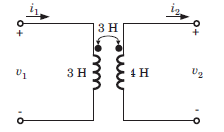i1  = 5sin 3t A and i2 = 3 cos 3t A. v2 = ?

Detailed Solution for Test: Magnetically Coupled Circuits - Question 1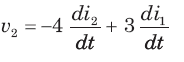=36 sin 3t + 45 cos 3t = 9(4sin 3t + 5cos 3t)  V

Test: Magnetically Coupled Circuits - Question 2

### In the circuit shown in fig. if current i1 = 5 cos (500t - 20°) mA and i2 = 20 cos (500t - 20°) mA, the total energy stored in system at t = 0 is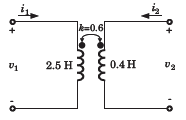Detailed Solution for Test: Magnetically Coupled Circuits - Question 2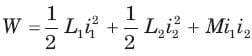At t = 0, i1 = 4 cos (-20°) = 4.7 mA
i2 = 20 cos (-20°) = 18.8 mA,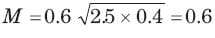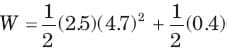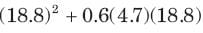= 151.3 μJ

Test: Magnetically Coupled Circuits - Question 3

### Leq  = ?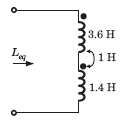Detailed Solution for Test: Magnetically Coupled Circuits - Question 3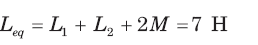Test: Magnetically Coupled Circuits - Question 4

Leq = ?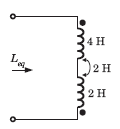Detailed Solution for Test: Magnetically Coupled Circuits - Question 4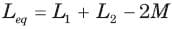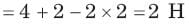Test: Magnetically Coupled Circuits - Question 5

Leq = ?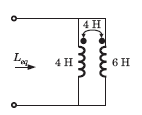Detailed Solution for Test: Magnetically Coupled Circuits - Question 5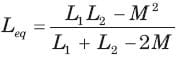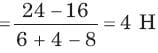Test: Magnetically Coupled Circuits - Question 6

Leq = ?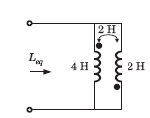Detailed Solution for Test: Magnetically Coupled Circuits - Question 6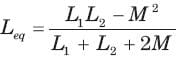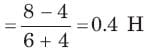Test: Magnetically Coupled Circuits - Question 7

The equivalent inductance of a pair of a coupled inductor in various configuration are
(a) 7 H after series adding connection
(b) 1.8 H after series opposing connection
(c) 0.5 H after parallel connection with dotted terminal connected together.

The value of L1 L2 , and M are

Detailed Solution for Test: Magnetically Coupled Circuits - Question 7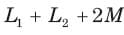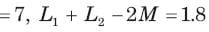⇒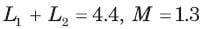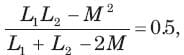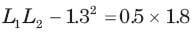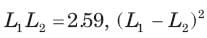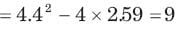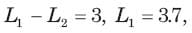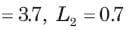Test: Magnetically Coupled Circuits - Question 8

Leq = ?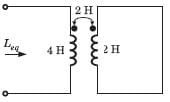Detailed Solution for Test: Magnetically Coupled Circuits - Question 8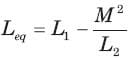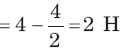Test: Magnetically Coupled Circuits - Question 9

Leq = ?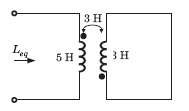Detailed Solution for Test: Magnetically Coupled Circuits - Question 9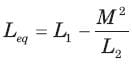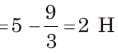Test: Magnetically Coupled Circuits - Question 10

Leq = ?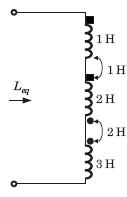Detailed Solution for Test: Magnetically Coupled Circuits - Question 10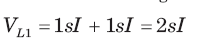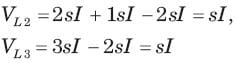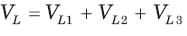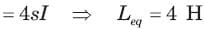Test: Magnetically Coupled Circuits - Question 11

Leq = ?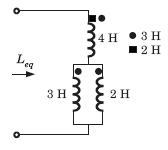Detailed Solution for Test: Magnetically Coupled Circuits - Question 11

Let I1 be the current through 4 H inductor and I2 and I3 be the current through 3 H, and 2 H inductor respectively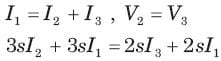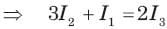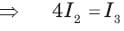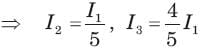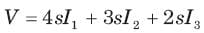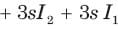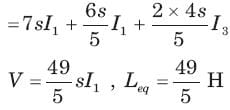Test: Magnetically Coupled Circuits - Question 12

Consider the circuit shown in fig.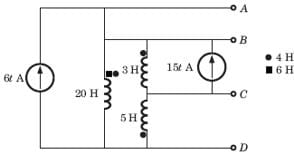The voltage VAG of terminal AD is

Detailed Solution for Test: Magnetically Coupled Circuits - Question 12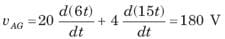Test: Magnetically Coupled Circuits - Question 13

Consider the circuit shown in fig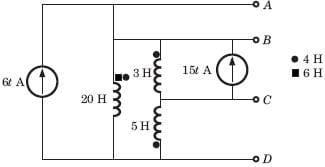The voltage vBG of terminal BD is

Detailed Solution for Test: Magnetically Coupled Circuits - Question 13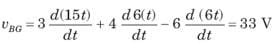Test: Magnetically Coupled Circuits - Question 14

Consider the circuit shown in fig.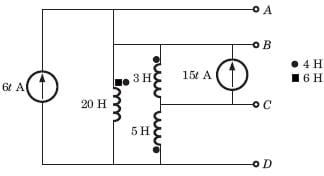The voltage vGC of terminal CD is

Detailed Solution for Test: Magnetically Coupled Circuits - Question 14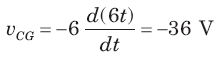Test: Magnetically Coupled Circuits - Question 15

In the circuit of fig. the  ω =2 rad/s. The resonance occurs when C is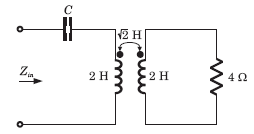Detailed Solution for Test: Magnetically Coupled Circuits - Question 15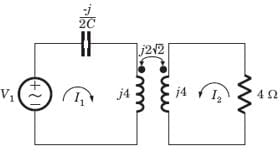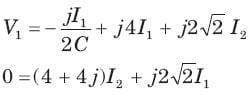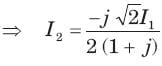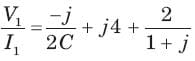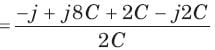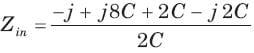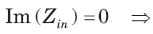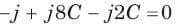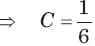Test: Magnetically Coupled Circuits - Question 16

In the circuit of fig, the voltage gain is zero at ω= 333.33 rad/s.

The value of C is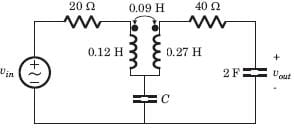Detailed Solution for Test: Magnetically Coupled Circuits - Question 16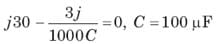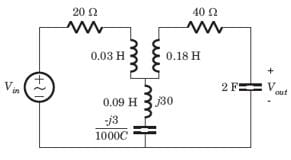Test: Magnetically Coupled Circuits - Question 17

In the circuit of fig. at ω = 333.33 rad/s, the voltage gain vout / vin  is zero. The value of C is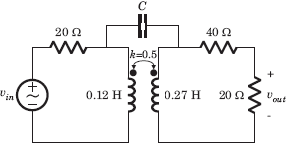Detailed Solution for Test: Magnetically Coupled Circuits - Question 17

The π  equivalent circuit of coupled coil is shown in fig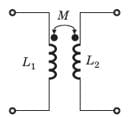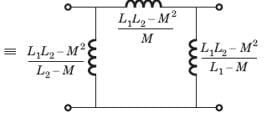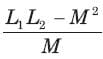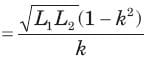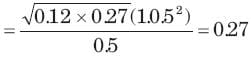Output is zero if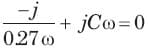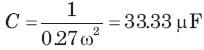Test: Magnetically Coupled Circuits - Question 18

The evenin equivalent at terminal ab for the network shown in fig. is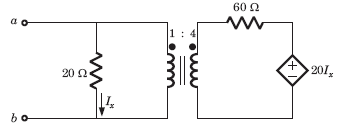Detailed Solution for Test: Magnetically Coupled Circuits - Question 18

Applying 1 V test source at ab terminal,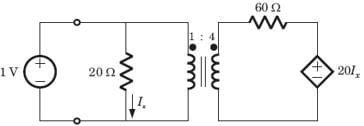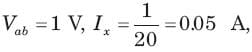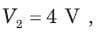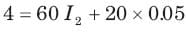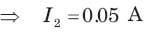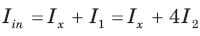= 0.25 A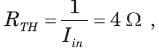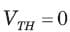Test: Magnetically Coupled Circuits - Question 19

In the circuit of fig. the maximum power delivered to RL is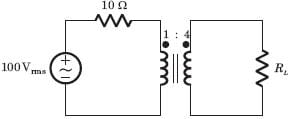Detailed Solution for Test: Magnetically Coupled Circuits - Question 19

Impedance seen by RL = 10 x 42 = 160 Ω
For maximum poer RL = 160Ω, Zo = 10Ω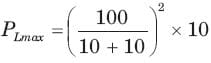= 250 W

Test: Magnetically Coupled Circuits - Question 20

The average power delivered to the 8 Ω load in the circuit of fig. is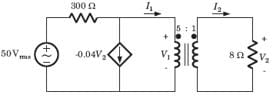Detailed Solution for Test: Magnetically Coupled Circuits - Question 20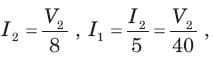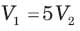## RRB JE for Electrical Engineering

2 videos|3 docs|75 tests
 Use Code STAYHOME200 and get INR 200 additional OFF Use Coupon Code
Information about Test: Magnetically Coupled Circuits Page
In this test you can find the Exam questions for Test: Magnetically Coupled Circuits solved & explained in the simplest way possible. Besides giving Questions and answers for Test: Magnetically Coupled Circuits, EduRev gives you an ample number of Online tests for practice

## RRB JE for Electrical Engineering

2 videos|3 docs|75 tests